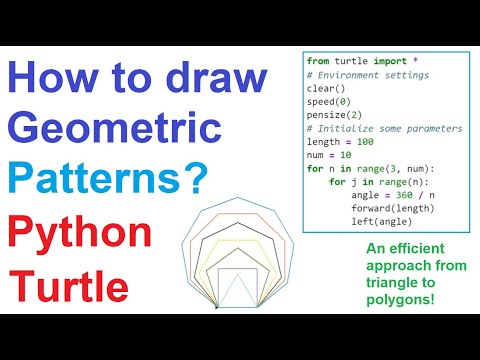# How to Draw Geometric Patterns with Python Turtle #7This tutorial presents drawing geometric patterns with Python Turtle graphics. First, an algorithm, drawing equilateral triangle-polygons, will be presented.

Lec-7: How to Draw Geometric Patterns with Python Turtle

This tutorial presents drawing geometric patterns with Python Turtle graphics. First, an algorithm, drawing equilateral triangle-polygons, will be presented. Then, an efficient Python code will be written based on the presented algorithm.

## top 30 Python Tips and Tricks for Beginners

In this post, we'll learn top 30 Python Tips and Tricks for Beginners